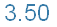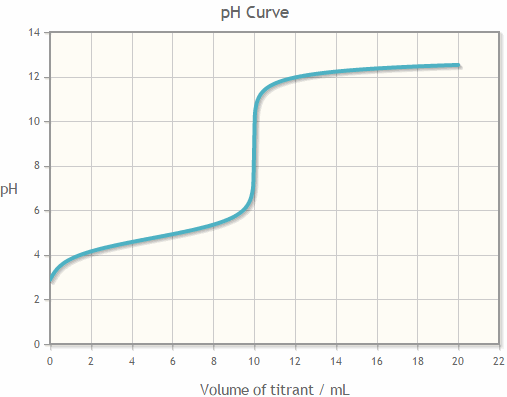# pH calculator## pH mixture

calculates the pH of a mixture

Example 1

Mixture of solutions:

• 100 mL of 0.1M NaOH
• 100 mL of H3PO4 0.1 M
• 100 mL of CH3COOH 0.1 M

pH of the resulting solution =Example 2

Mixture of solutions:

• 50 mL of NH3 0.2 M
• 100 mL of NH4Cl 0.1 M

pH of the resulting solution =* M = molar (mol/L)

## Titration Curves

traces the pH curve as a function of added titrant volume (titration)

Example 3

Titration of a solution of CH3COOH 0.1 M with 0.1 M NaOH